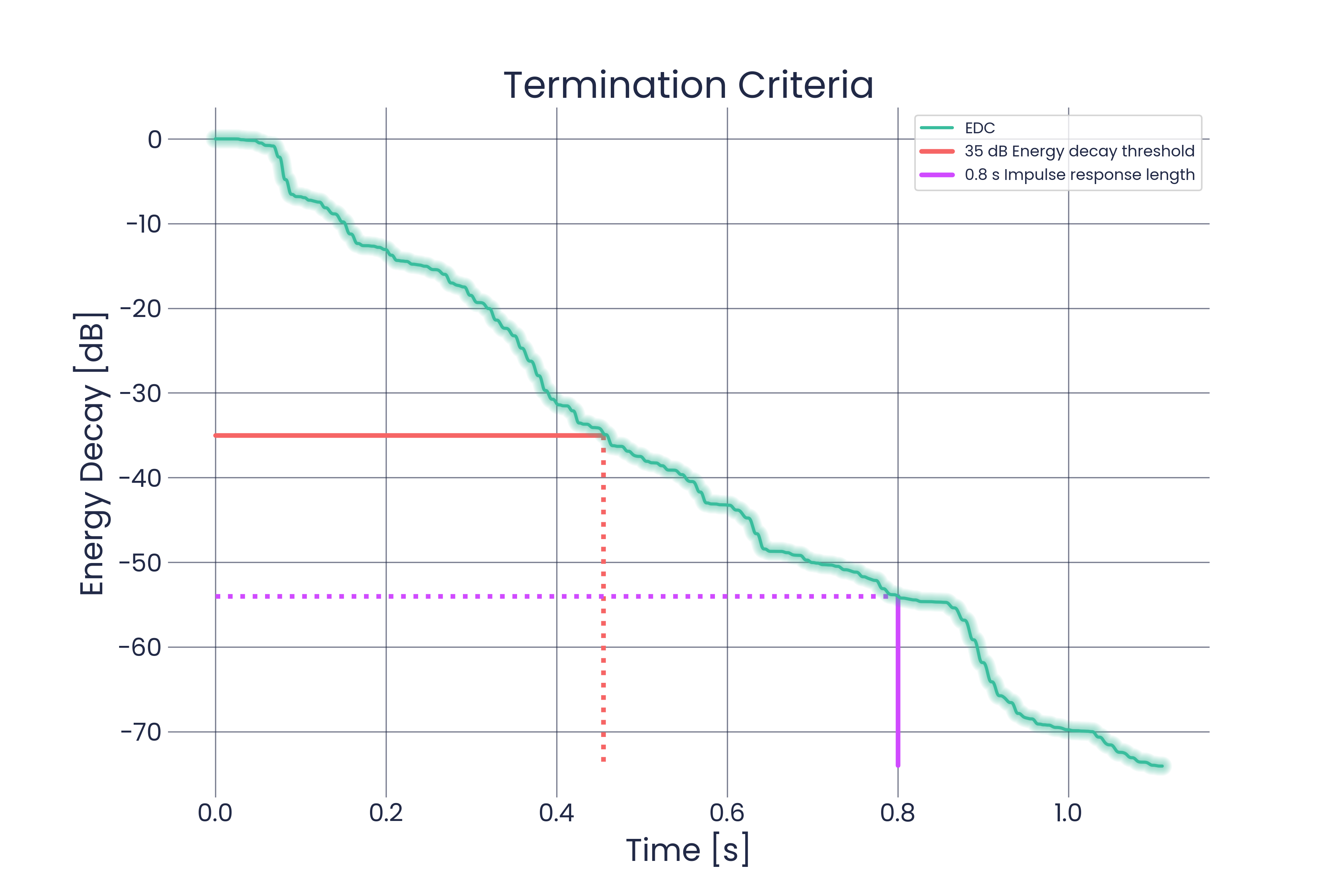# Termination Criteria

When a simulation is initialized, it needs to be clear when the simulation is supposed to be stopped. Treble offers the user to choose between two different ways of defining when the simulations should be stopped.

## Impulse Response Length​

One option for a termination criterion is the impulse response (IR) length. In that case the final time is chosen beforehand and the solvers will return their results when they have simulated an IR for the number of seconds that it was asked to. A potential issue here is that it can be hard to have an intuition for how long the IR needs to be in order for all of the information of interest to be contained in the IR. A good way is to base it on the Sabine equation

$T_{60} = \frac{0.161 V}{\sum_iS_i\alpha_i}$

where $V$ is the volume of the room and $S_i$ and $\alpha_i$ are the surface area and absorption of surface $i$, respectively.

The Sabine equation gives a decent estimate but is not fully reliable. Treble bases its suggested IR length on the Sabine equation, including safety factors based on our experience. The suggested IR length is usually long enough for computing the bulk of the acoustic parameters but it is not guaranteed that it will be

## Energy Decay Threshold​

Another way to define a termination criterion in Treble is the energy decay threshold. That essentially means how far down the Energy Decay Curve(EDC) needs to go down in dB until the solvers are stopped. This has to happen for every receiver included in the simulation. The default value here is 35 dB which allows for the calculation of both T20 and T30 reverberation times. If the user is interested in these acoustic parameters, inputting an energy decay threshold is a more robust way to guarantee that they will be computable from the simulation. They will also be computed if the IR length is used as a termination criterion, but only if the energy decays by at least 25 dB (T20) or 35 dB (T30) during the simulation time.

The energy decay threshold is not dependant on the size of the rooms or their absorption properties and can thus often be an easier parameter to develop an intution for.

## Overview​The figure shows the different termination criteria. In red, the energy decay facilitates the termination resulting in the simulation terminating after 0.45 s. In purple, the simulated time is what enforces termination, resulting in the simulation terminating after energy had dropped by 54 dB.

The figure does not include the buffer times we include in our computation of the acoustic parameters, but this is all accounted for in the solvers.